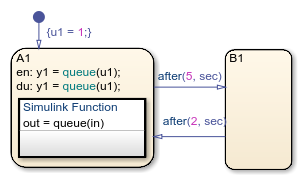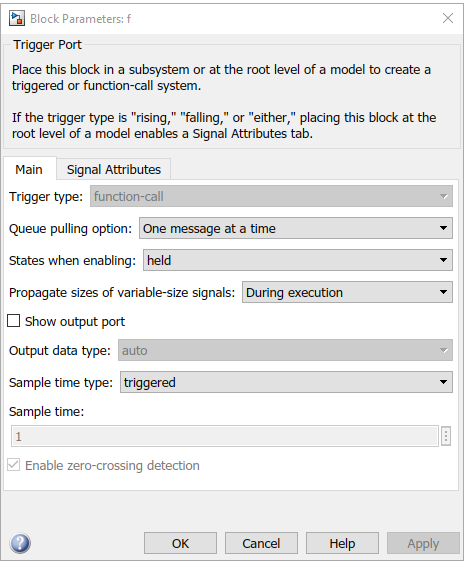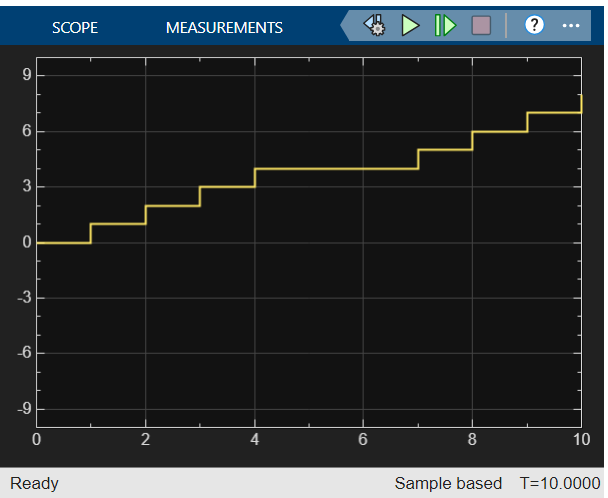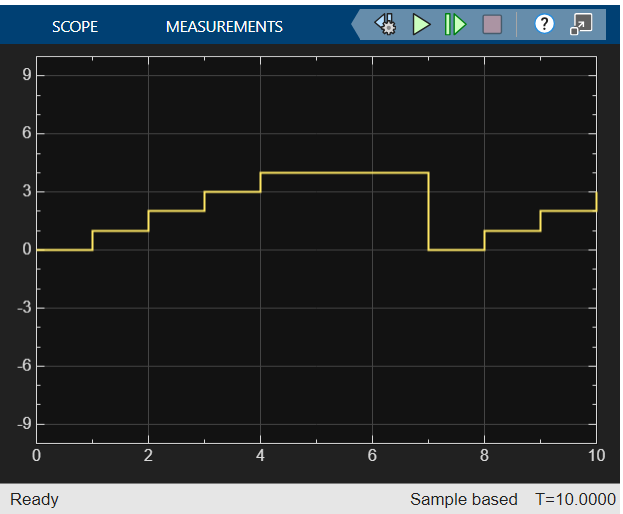Bind a Simulink Function to a State

Simulink® functions are not supported in standalone Stateflow® charts in MATLAB®.

When a Simulink function resides inside a state, the function binds to that state. Binding results in the following behavior:

• Function calls can occur only in state actions and on transitions within the state and its substates.

• When the state is entered, the function is enabled.

• When the state is exited, the function is disabled.

For example, the following Stateflow chart shows a Simulink function that binds to a state.Since the function queue resides in state A1, the function binds to state A1.

• State A1 and its substates A2 and A3 can call the function queue, but state B1 cannot.

• When state A1 is entered, queue is enabled.

• When state A1 is exited, queue is disabled.

Control Subsystem Variables When the Simulink Function Is Disabled

If a Simulink function binds to a state, you can hold the subsystem variables at their values from the previous execution or reset the variables to their initial values. To choose the desired behavior for your subsystem, follow these steps:

1. In the Simulink function, double-click the trigger port to open the Block Parameters dialog box.

2. Select an option for States when enabling.

OptionDescription
heldHolds the values of the subsystem variables from the previous execution
resetResets the subsystem variables to their initial values

Binding a Simulink Function to a State

This example shows how a Simulink function behaves when bound to a state.The function queue contains a block diagram that increments a counter by 1 each time the function executes.The Block Parameters dialog box for the trigger port appears as follows.In the dialog box, setting Sample time type to periodic enables the Sample time field, which defaults to 1. These settings tell the function to execute for each time step specified in the Sample time field while the function is enabled.

If you use a fixed-step solver, the value in the Sample time field must be an integer multiple of the fixed-step size. This restriction does not apply to variable-step solvers. For more information, see Compare Solvers (Simulink).

Simulation Behavior of the ChartWhen you simulate the chart, the following actions occur.

1. The chart takes the default transition to state A1, and the local data u1 is set to 1.

2. When A1 is entered, the function queue is enabled.

3. Function calls to queue occur until the condition after(5, sec) is true.

4. Once the condition is true, the transition from state A1 to B1 occurs.

5. When A1 is exited, the function queue is disabled.

6. After two more seconds pass, the transition from B1 to A1 occurs.

7. Steps 2 through 6 repeat until the simulation ends.

Function Behavior When Variables Are Held

If you set States when enabling to held, the output y1 is as follows.When state A1 becomes inactive at t = 5, the Simulink function holds the counter value. When A1 is active again at t = 7, the counter has the same value as it did at t = 5. Therefore, the output y1 continues to increment over time.

Function Behavior When Variables Are Reset

If you set States when enabling to reset, the output y1 is as follows.When state A1 becomes inactive at t = 5, the Simulink function does not hold the counter value. When A1 is active again at t = 7, the counter resets to zero. Therefore, the output y1 resets too.

Stateflow DocumentationGet trial now